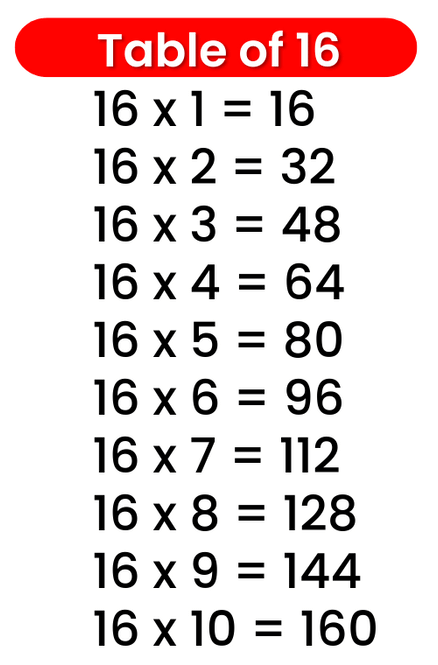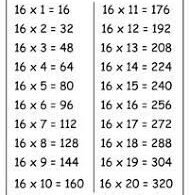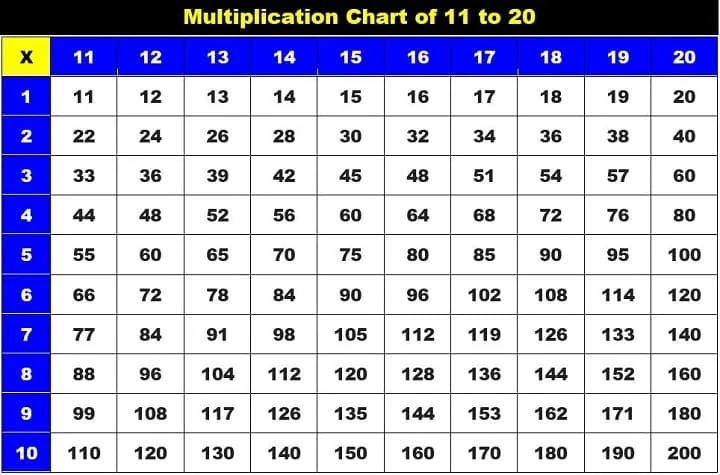# Times Table of 16, 16 ka table

## Times Table of 16, 16 ka table

Maths Multiplication Table of 16, Times Table of 16, 16 ka table, 16 ki Table Chart

16 ×‌ 1 = 16
16 ×‌ 2 = 32
16 ×‌ 3 = 48
16 ×‌ 4 = 64
16 ×‌ 5 = 80
16 ×‌ 6 = 96
16 ×‌ 7 = 112
16 ×‌ 8 = 128
16 ×‌ 9 = 144
16 ×‌ 10 = 160

## 16 ka table### Multiplication Tables of twelve upto 20### Times Table of 16 chart